﻿Random Method (Double, Double)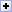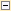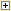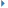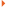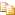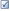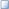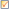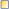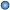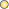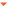Fills selected pixels with random values (Gaussian distribution).

Random values are mutiplied by 255 and truncated to the range 0..255.

Namespace: HiComponents.IEvolution
Assembly: IEvolution2 (in IEvolution2.dll) Version: 10.0.0.0

#Syntax

 C# Visual Basic Visual C++
```public void Random(
double mean,
double stdDev
)```
```Public Sub Random ( _
mean As Double, _
stdDev As Double _
)```
```public:
void Random(
double mean,
double stdDev
)```

#### Parameters

mean
Double
Mean value. 0.5 = gray (128).
stdDev
Double